IntroductionIntroduction# Surface Area of Square Pyramids

INTRO
The surface area of a 3D shape is equal to the sum of the areas of all the faces (sides) of that shape. A helpful way to think about it is:
surface area = sum of face areas
We can also think about surface area in terms of gifts! The surface area is like the minimum amount of wrapping paper we need to prep a gift for its surprise reveal. 🤩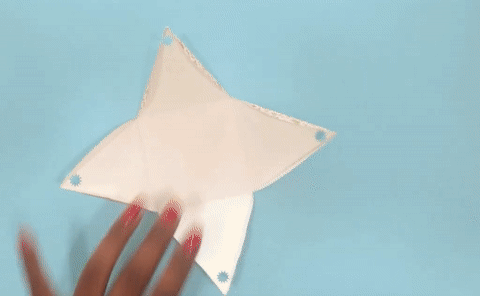If we were to unwrap a gift, the amount of wrapping would be equal to the surface area of the object it was covering.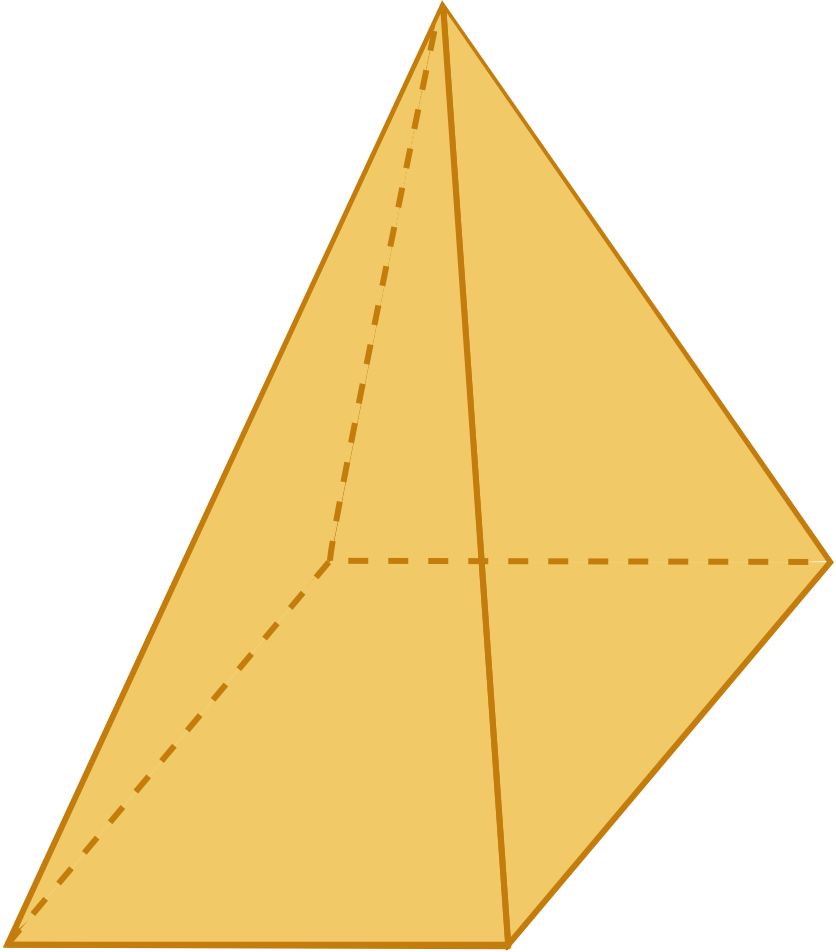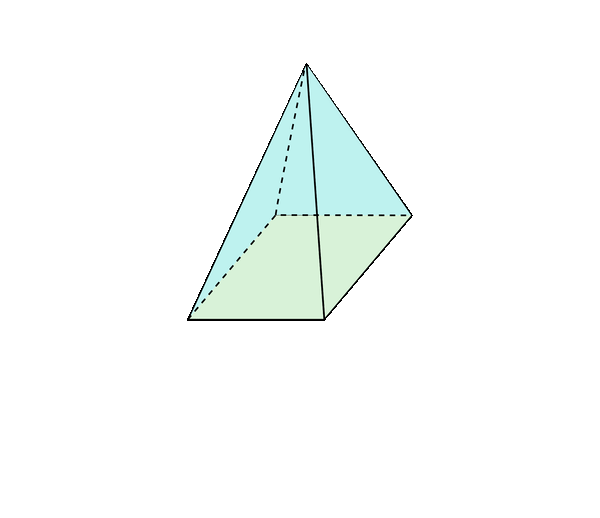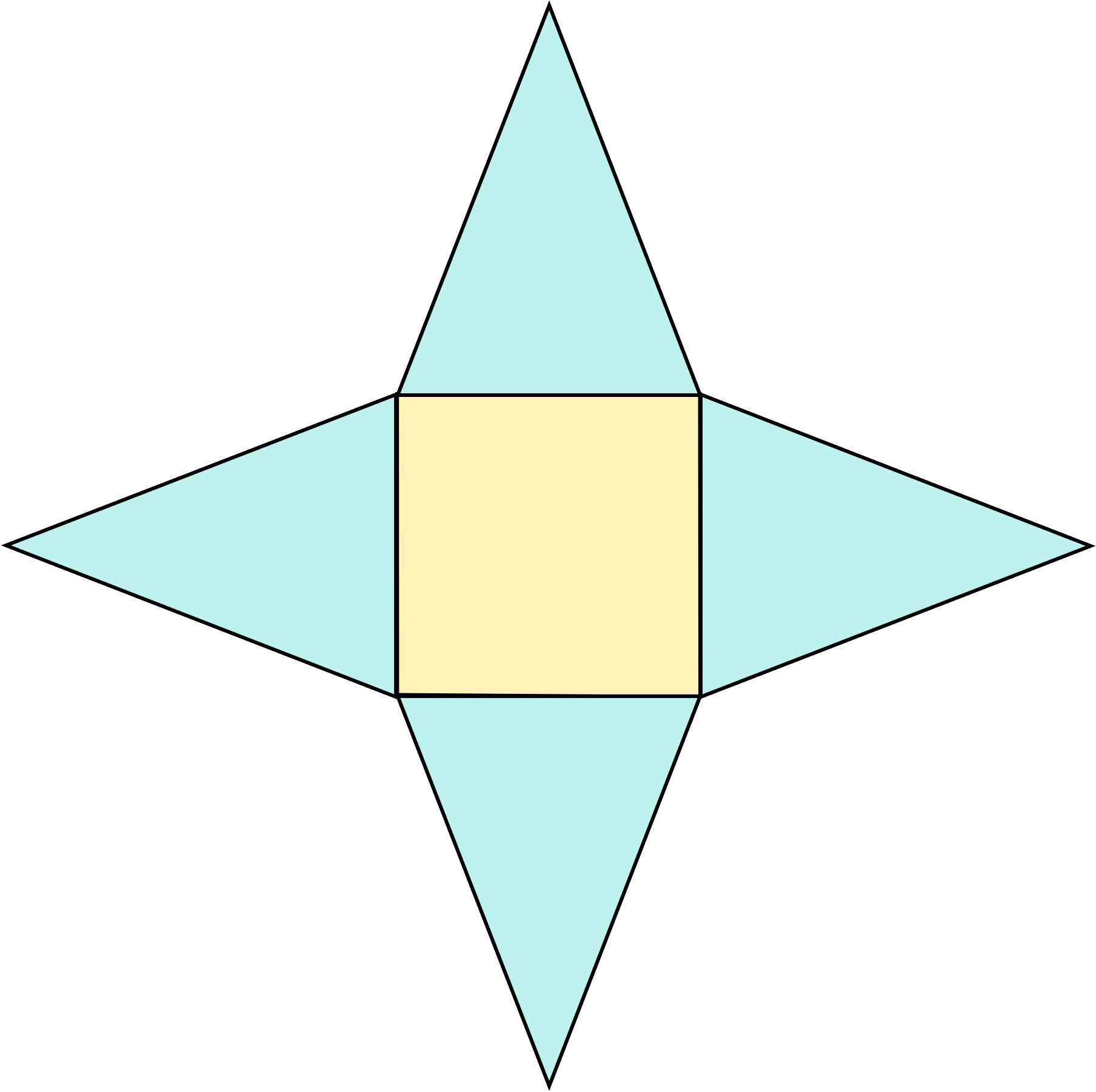When we “unwrap” a 3D shape, we get what we call a net. A surface area net shows us the different 2D shapes that make up the faces of the 3D shape.
We can then find the area of each face and then add them all together to find the total surface area.

### What is lateral surface area?

When working with pyramids, cylinders, and cones, you may also be asked to find the lateral surface area.
Lateral surface area is simply the total surface area of an object minus the area of its base(s).
Check out our
Calculator
or explore our
Lesson
and
Practice

CALCULATOR

## Surface Area of Square Pyramids Calculator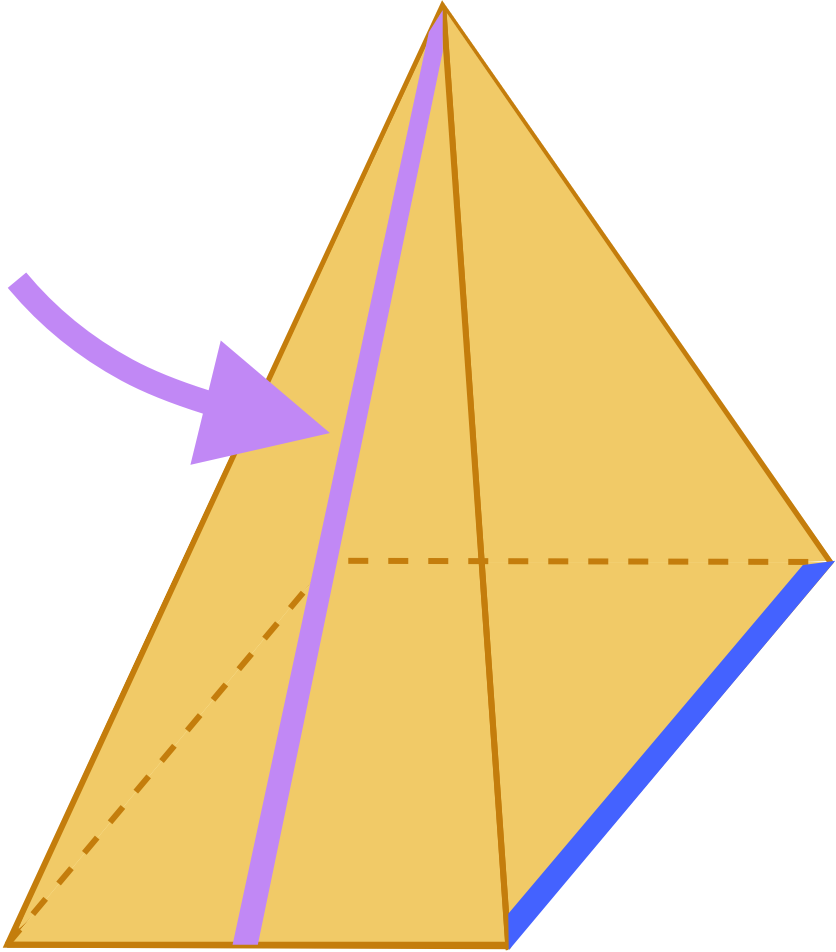Remember: is the slanted height of the pyramid!

KEY STEPS

## How to Find Surface Area of Square Pyramids

### Step 1. Unwrap the cone.### Step 2. Calculate the area of each part of the net that makes up the square pyramid.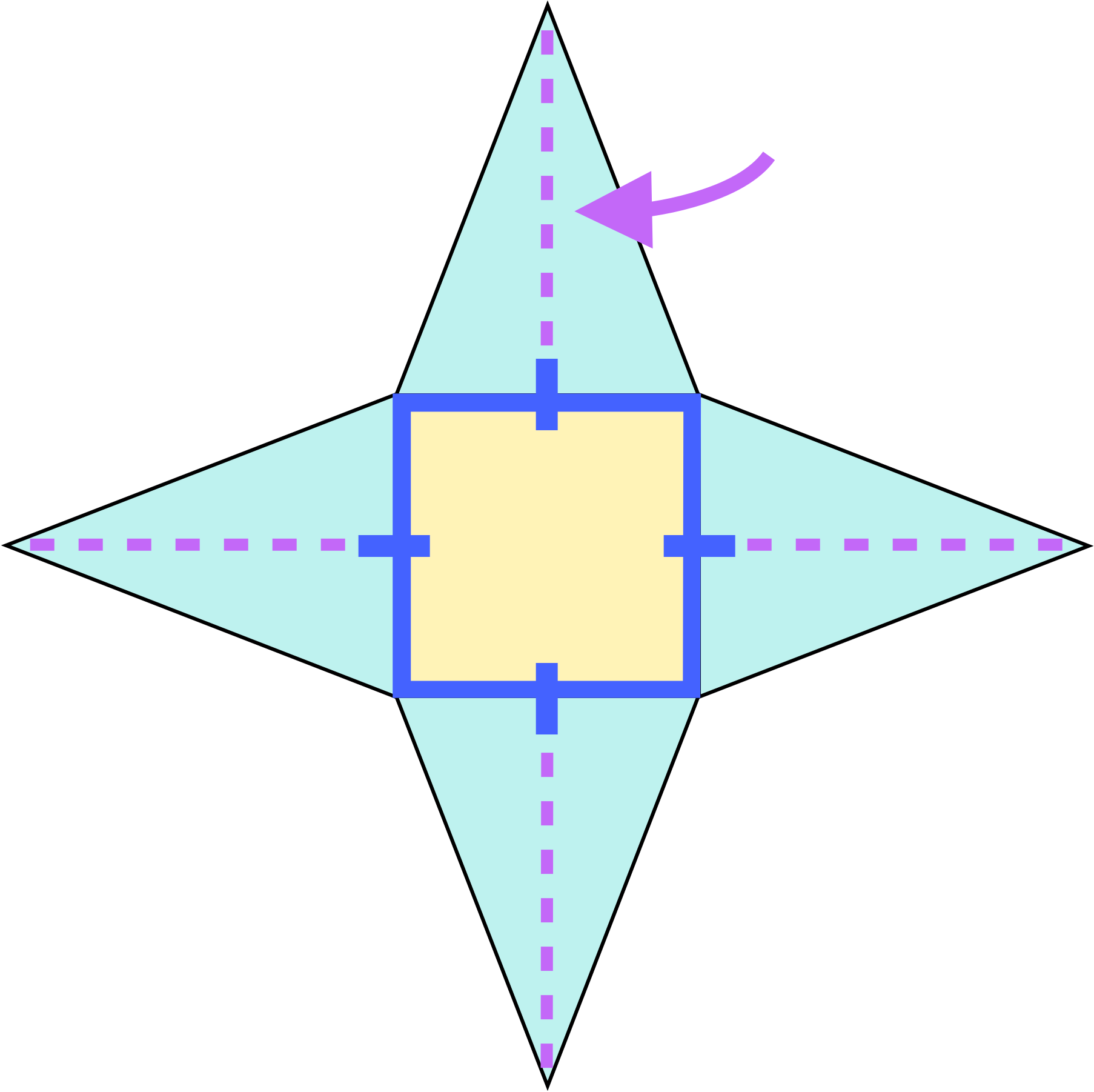Square Triangles

### Step 3. Add up the areas of the shapes that make up the square pyramid.

LESSON
Surface Area of Square Pyramids

## Surface Area of Square Pyramids

The surface area of a square pyramid is equal to the sum of the areas of all the faces (sides and base) of the pyramid.
If we unwrap a square pyramid, we can see that the net is made up of square and identical triangles.So, we need to first find the area of one of the triangles (). Then, we multiply it by and add it to the area of the square () to get the total surface area.Notice that the side length of the square base is the same as the base of the triangle, and the slanted height of the pyramid is the height of the triangle 😮.

### What about the lateral surface area?

If we wanted to find the lateral surface area of the square pyramid, we would exclude the area of the square base:
Now let's unwrap some cones to practice finding their surface area!
PRACTICE
Surface Area of Square Pyramids

## Practice: Surface Area of Square Pyramids

Question 1 of 5: Find the surface area of this square pyramid:### Step 1. Unwrap the square pyramid.CONCLUSION
Nice work, look at you go! Thanks for checking out this lesson ☺️🙏. Where to next?Leave Feedback